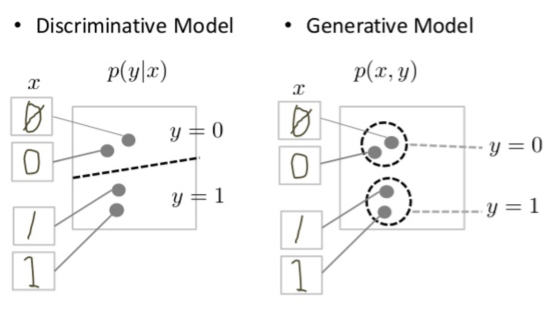# Background: What is a Generative Model?

What does "generative" mean in the name "Generative Adversarial Network"? "Generative" describes a class of statistical models that contrasts with discriminative models.

Informally:

• Generative models can generate new data instances.
• Discriminative models discriminate between different kinds of data instances.

A generative model could generate new photos of animals that look like real animals, while a discriminative model could tell a dog from a cat. GANs are just one kind of generative model.

More formally, given a set of data instances X and a set of labels Y:

• Generative models capture the joint probability p(X, Y), or just p(X) if there are no labels.
• Discriminative models capture the conditional probability p(Y | X).

A generative model includes the distribution of the data itself, and tells you how likely a given example is. For example, models that predict the next word in a sequence are typically generative models (usually much simpler than GANs) because they can assign a probability to a sequence of words.

A discriminative model ignores the question of whether a given instance is likely, and just tells you how likely a label is to apply to the instance.

Note that this is a very general definition. There are many kinds of generative model. GANs are just one kind of generative model.

## Modeling Probabilities

Neither kind of model has to return a number representing a probability. You can model the distribution of data by imitating that distribution.

For example, a discriminative classifier like a decision tree can label an instance without assigning a probability to that label. Such a classifier would still be a model because the distribution of all predicted labels would model the real distribution of labels in the data.

Similarly, a generative model can model a distribution by producing convincing "fake" data that looks like it's drawn from that distribution.

## Generative Models Are Hard

Generative models tackle a more difficult task than analogous discriminative models. Generative models have to model more.

A generative model for images might capture correlations like "things that look like boats are probably going to appear near things that look like water" and "eyes are unlikely to appear on foreheads." These are very complicated distributions.

In contrast, a discriminative model might learn the difference between "sailboat" or "not sailboat" by just looking for a few tell-tale patterns. It could ignore many of the correlations that the generative model must get right.

Discriminative models try to draw boundaries in the data space, while generative models try to model how data is placed throughout the space. For example, the following diagram shows discriminative and generative models of handwritten digits:Figure 1: Discriminative and generative models of handwritten digits.

The discriminative model tries to tell the difference between handwritten 0's and 1's by drawing a line in the data space. If it gets the line right, it can distinguish 0's from 1's without ever having to model exactly where the instances are placed in the data space on either side of the line.

In contrast, the generative model tries to produce convincing 1's and 0's by generating digits that fall close to their real counterparts in the data space. It has to model the distribution throughout the data space.

GANs offer an effective way to train such rich models to resemble a real distribution. To understand how they work we'll need to understand the basic structure of a GAN.

## Check Your Understanding: Generative vs. Discriminative Models

You have IQ scores for 1000 people. You model the distribution of IQ scores with the following procedure:
1. Roll three six-sided dice.
2. Multiply the roll by a constant w.
3. Repeat 100 times and take the average of all the results.
You try different values for w until the result of your procedure equals the average of the real IQ scores. Is your model a generative model or a discriminative model?
Generative model
Correct: with every roll you are effectively generating the IQ of an imaginary person. Furthermore, your generative model captures the fact that IQ scores are distributed normally (that is, on a bell curve).
Discriminative model
Incorrect: an analogous discriminative model would try to discriminate between different kinds of IQ scores. For example, a discriminative model might try to classify an IQ as fake or real.
Not enough information to tell.
This model does indeed fit the definition of one of our two kinds of models.
A model returns a probability when you give it a data instance. Is this model a generative model or a discriminative model?
Generative model
A generative model can estimate the probability of the instance, and also the probability of a class label.
Discriminative model
A discriminative model can estimate the probability that an instance belongs to a class.
Not enough information to tell.
Both generative and discriminative models can estimate probabilities (but they don't have to).
[{ "type": "thumb-down", "id": "missingTheInformationINeed", "label":"Missing the information I need" },{ "type": "thumb-down", "id": "tooComplicatedTooManySteps", "label":"Too complicated / too many steps" },{ "type": "thumb-down", "id": "outOfDate", "label":"Out of date" },{ "type": "thumb-down", "id": "samplesCodeIssue", "label":"Samples/Code issue" },{ "type": "thumb-down", "id": "otherDown", "label":"Other" }]
[{ "type": "thumb-up", "id": "easyToUnderstand", "label":"Easy to understand" },{ "type": "thumb-up", "id": "solvedMyProblem", "label":"Solved my problem" },{ "type": "thumb-up", "id": "otherUp", "label":"Other" }]